# Damped Harmonic OscillatorDamping coefficient
 Undamped oscillator
 Driven oscillator

The Newton's 2nd Law motion equation is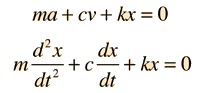This is in the form of a homogeneous second order differential equation and has a solution of the form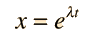Substituting this form gives an auxiliary equation for λ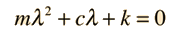The roots of the quadratic auxiliary equation areThe three resulting cases for the damped oscillator areIndex

Periodic motion concepts

 HyperPhysics***** Mechanics R Nave
Go Back

# Damping Coefficient

When a damped oscillator is subject to a damping force which is linearly dependent upon the velocity, such as viscous damping, the oscillation will have exponential decay terms which depend upon a damping coefficient. If the damping force is of the formthen the damping coefficient is given by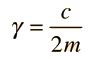This will seem logical when you note that the damping force is proportional to c, but its influence inversely proportional to the mass of the oscillator.

Index

Periodic motion concepts

 HyperPhysics***** Mechanics R Nave
Go Back

# Underdamped Oscillator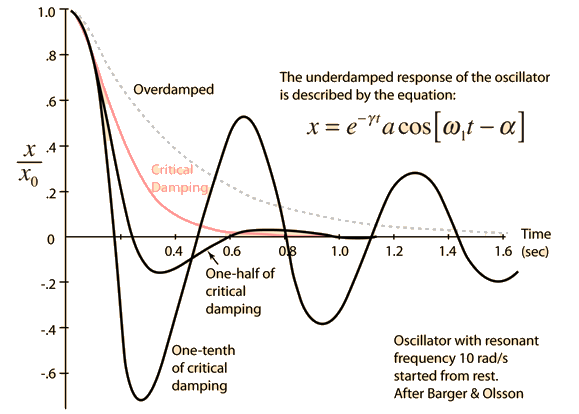For any value of the damping coefficient γ less than the critical damping factor the mass will overshoot the zero point and oscillate about x=0. The behavior is shown for one-half and one-tenth of the critical damping factor. Also shown is an example of the overdamped case with twice the critical damping factor.

Note that these examples are for the same specific initial conditions, i.e., a release from rest at a position x0. For other initial conditions, the curves would look different, but the the behavior with time would still decay according to the damping factor.

 Damped Oscillator Further details for underdamped case
Index

Periodic motion concepts

Reference

Barger & Olsson

 HyperPhysics***** Mechanics R Nave
Go Back

# Underdamped Oscillator

When a damped oscillator is underdamped, it approaches zero faster than in the case of critical damping, but oscillates about that zero.The equation is that of an exponentially decaying sinusoid.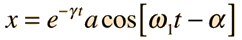The damping coefficient is less than the undamped resonant frequency . The sinusoid frequency is given bybut the motion is not strictly periodic.
Index

Periodic motion concepts

 HyperPhysics***** Mechanics R Nave
Go Back